Search IntMath
Close

# Triangles

A triangle is a polygon with three edges and three vertices. It is one of the basic shapes in geometry. A triangle with vertices A, B, and C denoted △ABC is given below: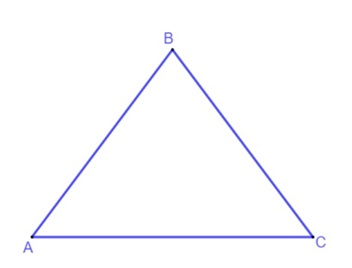In the triangle above, the edges are AB,  BC and AC. The angles are ∠A, ∠B and ∠C. Triangles can be classified according to the side lengths or its interior angles.

## The Three Types of Triangles (Classified by Angles)

When classified by their angles, there are three main types of triangles: acute, right, and obtuse. An acute triangle has all three angles measuring less than 90°, a right triangle has one angle measuring 90°, and an obtuse triangle has one angle measuring more than 90°.

## Acute Triangle

An acute triangle is a triangle with all three angles measuring less than 90°. In other words, all the angles of an acute triangle are acute angles.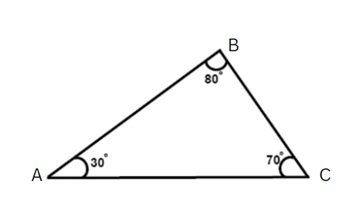The above figure shows an acute angled triangle ABC in which the angles A, B and C are acute angles less than 90°.

## Right Triangle

A right triangle is a triangle in which one angle measures 90° and the other two angles measure less than 90°. In a right angled triangle, the side opposite to the right angle is called the hypotenuse while the other two sides are called legs or catheti (singular: cathetus). The hypotenuse is always the longest side while the other two sides are shorter. The below figure shows a right angled triangle ABC in which angle C measures 90°.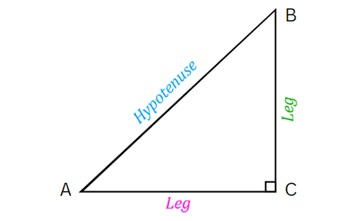## Obtuse Triangle

An obtuse triangle is a triangle with one angle measuring more than 90° while the other two angles measure less than 90° each.  In other words, an obtuse angled triangle has one obtuse angle and two acute angles. The below figure shows an obtuse angled triangle ABC in which angle B measures more than 90° while angles A and C measure less than 90° each.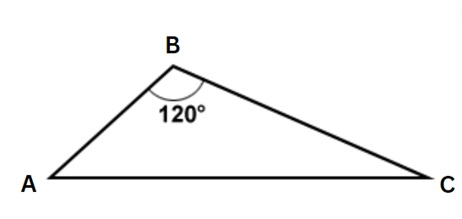## The Three Types of Triangles (Classified by Side Lengths)

When classified by side lengths, there are three main types of triangles: scalene, isosceles, and equilateral triangles.

## Scalene Triangle

In a scalene triangle, all the side lengths are of different measures and hence, all the angles are of different measures.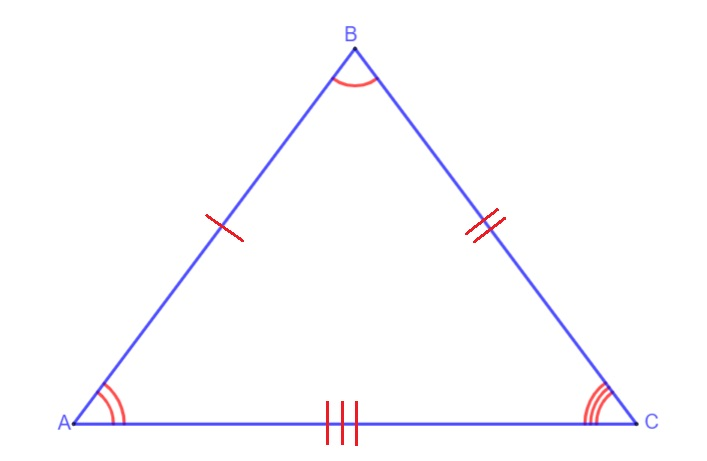The above figure shows a scalene triangle ABC in which the angles A, B and C are all of different measures.

## Isosceles Triangle

An isosceles triangle is a triangle with two of its side lengths equal in length. In an isosceles triangle, the angles at the base of the congruent sides are referred to as the base angles and are of equal measures.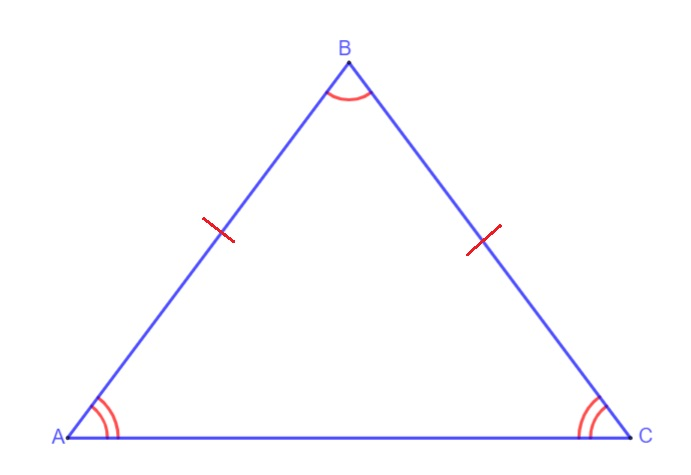In triangle ABC above, the equal side lengths are AB and BC. The base angles are A and C, therefore m∠A ≅ m∠B.

## Equilateral Triangle

An equilateral triangle is a triangle with all three sides equal in length. In an equilateral triangle, each of the interior angles has a measure of 60 degrees.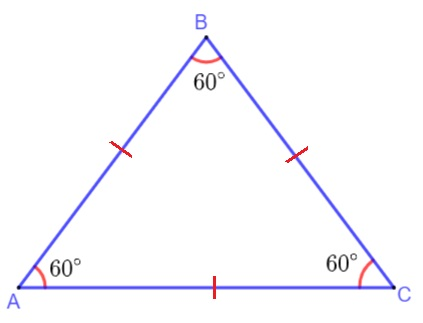All equilateral triangles are similar.

## Conclusion:

In general, there are 7 types of triangles when classified by a mixture of its angles and lengths. They are equilateral, right isosceles, obtuse isosceles, scalene, acute scalene, right scalene, and obtuse scalene.

There you have it! Next time you see a triangle, see if you can classify it by its angles!

## FAQ

What is a triangle in geometry?

A triangle is a polygon with three edges and three vertices. It is one of the basic shapes in geometry.

### Classified by its angles, what are the 3 types of triangle?

The three types of triangle are acute, right, and obtuse.

### Classified by its side lengths, what are the 3 types of triangle?

The three types of triangle are scalene, isosceles, and equilateral triangles.

### What are the 7 triangles?

The seven general types of triangles are the equilateral, right isosceles, obtuse isosceles, scalene, acute scalene, right scalene, and obtuse scalene.

## Problem SolverThis tool combines the power of mathematical computation engine that excels at solving mathematical formulas with the power of GPT large language models to parse and generate natural language. This creates math problem solver thats more accurate than ChatGPT, more flexible than a calculator, and faster answers than a human tutor. Learn More.